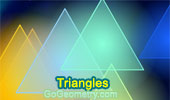# Triangles: Theorems and Problems 23Archimedes Arbelos and Square 2. Dynamic Geometry Software.
Step-by-Step construction, Manipulation, and animation.

Archimedes Arbelos and Square 1. Dynamic Geometry Software.
Step-by-Step construction, Manipulation, and animation.

Proposed Problem 211. 60 Degree Triangle, Equilateral triangles, Areas.

Proposed Problem 210. Triangle, Angles, Auxiliary Lines.

Proposed Problem 209. Triangle, Incircles, Inradius.

Proposed Problem 208. Triangle, Excircles, Angles, 360 degrees.